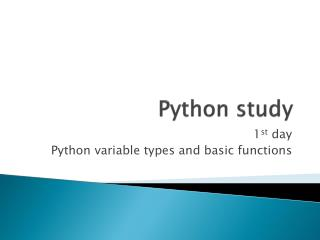DownloadDownload PresentationPython study

# Python study

Télécharger la présentation## Python study

- - - - - - - - - - - - - - - - - - - - - - - - - - - E N D - - - - - - - - - - - - - - - - - - - - - - - - - - -
##### Presentation Transcript

1. Python study 1st day Python variable types and basic functions

2. Homework check!

3. Variable • What is the Variable? • These three formula are same • although their variable names are different • We can set the variable names whatever you want • But! We have to know • In A = B, A is the name of variable and B is the value • It is called as variable setting • If you did not set variable, you cannot use them

4. Variable

5. Variable type • String type • All the characters are string type • ‘a’, ‘b’, ‘c’, ‘d’, ‘0’, ‘1’, ‘2’, ‘3’, ‘0.1’… • You have to use ‘’ or “” for string type • A : variable A • ‘A’ : string value A • Special character(\ = ₩) • ‘\n’ : newline character • ‘\t’ : tab • ‘\’’ : ‘ • ‘\”’ : “ • ‘\\’ : \

6. Variable type • Integer type • All the integers are integer type • 1, 2, 3, 4, 100, 72038, 900223 • Float type • Represent decimal number or fractional number • 1/3, 0.23, 1.8, 3.141592

7. Characteristics of Variable • Cannot use add between strand int type variable • ‘Crop’ + ‘ Genomics’ = ‘Crop Genomics’ • ‘Crop’ + ‘4555’ = ‘Crop4555’ • ‘880’ + ‘4555’ = ‘8804555’ • 880 + 4555 = 5435 • ‘880’ + 4555 = error • ‘Crop’ + 4555 = error • Between str and float also.

8. Characteristics of Variable • If you use float at least once, that variable will be float • 5/2 = 2 • 1+2 = 3 • 5.0/2 = 2.5 • 5/2.0 = 2.5 • 1.0+2 = 3.0

9. Characteristics of Variable • You can multiply string variable • 2*3 = 6 • ‘2’*3 = 222 • ‘hello’*3 = hellohellohello • Hello*3 vs. ‘Hello’*3

10. Characteristics of Variable • You can use these kind of symbols in integer and float type variable • +, -, *, / • //, %

11. Other variables • List • Dictionary

12. Other variables • List • Is set by [] • The list of other values or variable • List_a = [1,2,’a’,’b’,[a,b]] • List also can value of list • Can get empty value • List_b = []

13. Other variables • Dictionary • Is set by {} • Like a dictionary, had keys and values • Dic_a = {‘English’:‘영어’} →Dic_a[‘English’] = ‘영어’ • One key only have one value whatever, list, string, integer or dictionary • Usage) • Dic_amino_acid = {‘ATG’:‘Met’, ‘TGA:*’} • Dic_amino_acid = {}Dic_amino_acid[‘ATG’] = ‘Met’ • Key = [‘ATG’,’TGA’]value = [‘Met’, ‘*’]Dic_amino_acid = dict(zip(key,value))

14. Start python coding • vi filename.py • Python code files have .py as extension

15. Basic functions(함수) • What is the fuction(함수)? • Already set fuction(기능) by other programmer • Ex) print, if, for, open, etc.. • Print (standard output function) • Function for print something • Usage) • Print a • Print ‘a’ • Print ‘a’*3 • Print 3*4 • Print printwith newline character • Print ‘a\n’

16. Basic functions • Standard input functions • Input • For integer • Raw_input • For string • Usage) • A = input(“enter some integers”) • B = raw_input(“enter some words”)

17. Basic functions • If • For judgment • If conditional sentence were satisfied, some command were executed • If not, the other command were executed

18. Basic functions True False • If if … elif … else Status A Status B Status C

19. Basic functions • Functions for loop • For • Useful for limited loop • Usage) For variable_name in list_name: • range() • make list of integer • Ex) range(2) = [0,1] range(1,5) = [1,2,3,4] range(1,5,2) = [1,3] • len() • Calculate length • Ex) len(‘ABC’) = 3len([1,2]) = 2

20. Basic functions • Functions for loop • While • Useful for infinite loop • Usage while conditional_sentence: • If conditional sentence is true, loop are work. • While 1 mean always true, so it is infinite loop

21. Basic functions • break & continue • They are always used with if and loopfunctions • break • If conditional sentence is true, the loop will be terminated • continue • If conditional sentence is true, that element of loop will be passed

22. Homework • "This is sequence file"을 화면에 출력하시오.  • standard input으로(즉,화면에서) 임의의 문자열 입력받아 화면에 출력하시오.  • 두 정수를 standard input으로 입력받아 변수 a와 b에 저장하고 이들의 합을 변수 c에 저장한 후 결과를 출력하시오.  • 두 수를 입력하고 둘 중에 큰 수를 출력해주는 프로그램을 작성하시오.  • Standard input으로3-base codon을 입력받아서 아미노산으로 출력하시오.  • 4번의 문제에서 입력을 계속 받을 수 있도록 수정하시오.